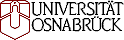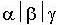# Complexity results for scheduling problems

### http://www.informatik.uni-osnabrueck.de/knust/class/

#### http://www.mathematik.uni-osnabrueck.de/research/OR/class/

Idea: Peter Brucker
Realization: Sigrid Knust

# Introduction

Lageweg et al. (1981), (1982) developed a computer program MSPCLASS for an automatic classification of scheduling problems. Based on the-classification scheme of Graham et al. (1979) it calculates problems which are
• maximal polynomially solvable: the hardest problems which are polynomially solvable, i.e. problems which are known to be polynomially solvable, but any harder cases are not known to be polynomially solvable,
• maximal pseudopolynomially solvable: the hardest problems which are known to be pseudopolynomially (but not polynomially) solvable,
• minimal NP-hard: the easiest problems which are NP-hard, i.e. problems which are known to be NP-hard, but any easier cases are not known to be NP-hard,
• minimal open: problems for which the complexity status is not known, but all easier cases are known to be polynomially solvable,
• maximal open: problems for which the complexity status is not known, but all harder cases are known to be NP-hard.

The input for MSPCLASS are problems which are known to be polynomially solvable or NP-hard and elementary reductions for scheduling problems.

The objective of these pages is to

• update complexity results
• extend the classification to new classes of scheduling problems.

For this purpose we developed a new computer program CLASS (Plaggenborg (1994)) and applied it to several classes of scheduling problems which are listed below. The used reduction graphs and obtained results can be found on the following pages. A * before an NP-hard problem denotes that the problem is NP-hard in the strong sense. Note that an arc A --> B in a reduction graph means a polynomial reduction from A to B only if the size of B is polynomially bounded by the size of A.
For notation we refer to Graham et al. (1979) and to Brucker (1998). In this book also most of the polynomial time algorithms can be found. All data are assumed to be integer.

We want to keep these pages as up to date as possible. If you have further results or any suggestions please contact us. Unpublished results should be sent to us as Latex or postscript files or as hardcopy.

Supported by the Deutsche Forschungsgemeinschaft, Project "Komplexe Maschinen-Schedulingprobleme''.

References:

• P. Brucker (1998): Scheduling algorithms, 2nd edition, Springer, Heidelberg.
• R.L. Graham, E.L. Lawler, J.K. Lenstra, A.H.G. Rinnooy Kan (1979): Optimization and approximation in deterministic sequencing and scheduling: a survey, Ann. Discrete Math. 4, 287-326.
• B.J. Lageweg, E.L. Lawler, J.K. Lenstra, A.H.G. Rinnooy Kan (1981): Computer aided complexity classification of deterministic scheduling problems, Report BM 138, Centre for Mathematics and Computer Science.
• B.J. Lageweg, E.L. Lawler, J.K. Lenstra, A.H.G. Rinnooy Kan (1982): Computer-aided complexity classification of combinatorial problems, Comm. ACM 25, 817-822.
• S. Plaggenborg (1994): Ein Algorithmus zur komplexitaetsmaessigen Klassifikation von Schedulingproblemen, Diplomarbeit Fachbereich Mathematik/Informatik, Universitaet Osnabrueck.

 Reduction Graph Complexity results Single machine problems [red. graph] [html] [pdf] Parallel machine problems without preemption [red. graph] [html] [pdf] Parallel machine problems with preemption [red. graph] [html] [pdf] Open-shop problems without preemption [red. graph] [html] [pdf] Open-shop problems with preemption [red. graph] [html] [pdf] Flow-shop problems without preemption [red. graph] [html] [pdf] Flow-shop problems with preemption [red. graph] [html] [pdf] Job-shop problems without preemption [red. graph] [html] [pdf] Job-shop problems with preemption [red. graph] [html] [pdf] Open-shop problems with nowait [red. graph] [html] [pdf] Flow-shop problems with nowait [red. graph] [html] [pdf] Job-shop problems with nowait [red. graph] [html] [pdf] Multiprocessor task problems with dedicated processors [red. graph] [html] [pdf] Multiprocessor task problems with dedicated processors and preemption [red. graph] [html] [pdf] Multiprocessor task problems with parallel processors [red. graph] [html] [pdf] Multiprocessor task problems with parallel processors and preemption [red. graph] [html] [pdf] Shop problems with multiprocessor tasks [red. graph] [html] [pdf] Multipurpose machine problems [red. graph] [html] [pdf] Shop problems with multipurpose machines [red. graph] [html] [pdf] Serial batching problems [red. graph] [html] [pdf] Parallel batching problems [red. graph] [html] [pdf] Single machine problems with non-negative time-lags [red. graph] [html] [pdf] Flow-shop problems with transportation delays [red. graph] [html] [pdf] Open-shop problems with transportation delays [red. graph] [html] [pdf] Flow-shop problems with transportation times and a single robot [red. graph] [html] [pdf] Parallel machine problems with a single server [red. graph] [html] [pdf] Flow-shop problems with a single server [red. graph] [html] [pdf]

Last update: 29.06.2009 (SK)